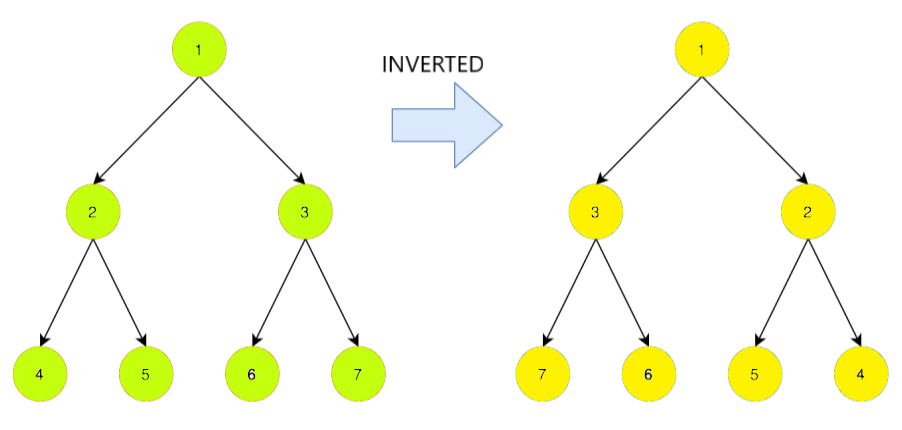# Invert a Binary Tree – Recursive and Iterative Approach in Java

In this article we have a look at another interesting problem related to binary tree. Given a binary tree we have to invert the tree and print it. We discuss different approaches to solve this problem along with their time and space complexities

The inversion of a binary tree or the invert of a binary tree means to convert the tree into it’s Mirror image. In simple terms, it is a tree whose left children and right children of all non-leaf nodes are swapped.

Note: The leaf nodes will also get interchanged. It is recommended to learn In-Order and Post Order traversal before proceeding with this problem.

Let us look at an example of a binary tree:In the above figure we see the sample tree and it’s inverted or mirror version.

## Approach 1 (Recursive Approach)

• We basically do a Post-Order Traversal of our tree starting from the root where we traverse for the left subtree first and then the right subtree before processing the root.
• At time of processing the root of each node we swap their left and right child respectively if their children are not null.
• We also check if the given root of binary tree is null or not. Then, we continue traversing for the left and right subtrees accordingly. If, we are at a leaf node it does not have any children for which we return null indicating that no swapping is required.
• To verify the tree is inverted or not we do the In-Order traversal of tree before executing the function and again print the In-Order traversal after doing inversion. You can verify the output using any traversal algorithm.

Now, let’s look at the implementation of above in code:

Output:

You can see the difference in output. The In-Order traversal for the given input tree displays output in sorted order and the In-order traversal of the Inverted tree gives output in reverse sorted order.

Time Complexity: We just traverse all the nodes present in tree so the time complexity will be O(n).

Space Complexity: If we consider the system stack space for each recursive call then complexity is O(n) otherwise it is O(1).

## Approach 2 (Iterative Approach)

The main idea is to do a Level Order Traversal of the tree using a Queue. We enqueue the root first into the Queue. While we traverse all the nodes at a particular level we swap the left child and right child of each node. After this, we add the left and right child child of the current node into our queue to continue the level order traversal.

Finally, we print the In-order traversal of the tree to verify the inverted tree.

Let us have a look at the implementation:

Output:

Time Complexity: It is same as recursive approach which require O(n) time as we traverse all nodes of tree.

Space Complexity: We use a Queue which stores all the nodes during execution so complexity is O(n).

That’s it for the article you can try and execute the above code. Feel free to ask your queries in the comment section.